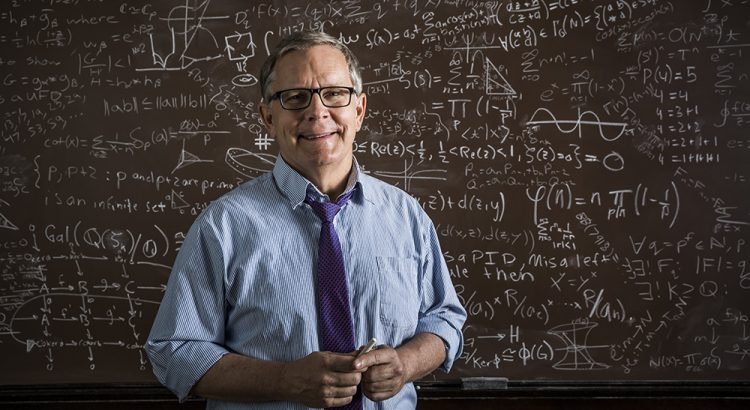# Mathematician

Mathematics is one of the oldest and most fundamental sciences. Mathematicians do research in fields such as logic, set theory, abstract algebra, number theory, geometry, topology, dynamical systems, combinatorics, game theory, probability, and statistics. Although math has numerous applications, mathematicians also find math interesting in its own right.

 Low End Salary:   \$56,200/yrMedian Salary:   \$111,110/yrHigh End Salary:   \$167,250/yr## Education:

A Ph.D. in mathematics is usually needed to work as a mathematician, although there are some opportunities for those with a master’s degree. There are also more job opportunities for those who have experience in a related field, such as computer science or engineering.

## Math Required:

College Algebra, Trigonometry, Calculus I, II, and III, Linear Algebra, Ordinary Differential Equations, Real Analysis, Abstract Algebra, Theory of Analysis, Complex Analysis. This is a very basic list; most mathematicians will study all the math they can and will study additional topics depending on their interests.

## When Math is Used:

Mathematicians use mathematical theory, computational techniques, algorithms, and the latest computer technology to solve economic, scientific, engineering, and business problems. There are two broad classes of mathematicians: those who work in theoretical mathematics and those who work in applied mathematics (although there's a lot of overlap).

## Potential Employers:

Many people are familiar with mathematicians in academia, but mathematicians also work in many other fields, including astronomy and space exploration, climate study, medicine, robitics, animated films, a wide range of businesses and even national security. Many mathematicians work for the Federal Government, with about 80 percent of those working for the U.S. Department of Defense. Other positions within the Federal Government include working for the National Institute of Standards and Technology (NIST) or the National Aeronautics and Space Administration (NASA). Other employers include technical consulting services and insurance carriers.

## Facts:

• The British mathematician, Alan Turing, is credited with helping to end World War II by cracking the Enigma Code • A mathematician is not strictly confined to manipulated formulas, numbers, or equations. In fact, mathematicians research new concepts and ideas.

## Citations:

• http://www.bls.gov/oco/ocos043.htm
• http://en.wikipedia.org/wiki/Mathematician
• http://www.po28.dial.pipex.com/maths/mathms.htm
• www.ams.org/notices/199806/microsoft.pdf
• www.maa.org/pubs/march07web.pdf
• http://www.bls.gov/oes/current/oes152021.htm